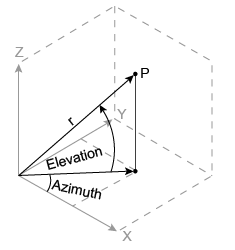# sph2cart

## 语法

``[x,y,z] = sph2cart(azimuth,elevation,r)``

## 说明

``[x,y,z] = sph2cart(azimuth,elevation,r)` 将球面坐标数组 `azimuth`、`elevation` 和 `r` 的对应元素转换为笛卡尔坐标，即 xyz 坐标。`

## 示例

`az = [0.7854 0.7854 -0.7854 -0.7854; 2.3562 2.3562 -2.3562 -2.3562]`
```az = 2×4 0.7854 0.7854 -0.7854 -0.7854 2.3562 2.3562 -2.3562 -2.3562 ```
`el = [0.6155 -0.6155 0.6155 -0.6155; 0.6155 -0.6155 0.6155 -0.6155]`
```el = 2×4 0.6155 -0.6155 0.6155 -0.6155 0.6155 -0.6155 0.6155 -0.6155 ```
`r = 1.7321*ones(2,4)`
```r = 2×4 1.7321 1.7321 1.7321 1.7321 1.7321 1.7321 1.7321 1.7321 ```
`[x,y,z] = sph2cart(az,el,r)`
```x = 2×4 1.0000 1.0000 1.0000 1.0000 -1.0000 -1.0000 -1.0000 -1.0000 ```
```y = 2×4 1.0000 1.0000 -1.0000 -1.0000 1.0000 1.0000 -1.0000 -1.0000 ```
```z = 2×4 1.0001 -1.0001 1.0001 -1.0001 1.0001 -1.0001 1.0001 -1.0001 ```

## 输入参数

`azimuth` 是在 x-y 平面上从 x 轴的正向测量的逆时针角度（以弧度为单位）。

`elevation` 是与 x-y 平面之间形成的仰角（以弧度为单位）。

`r` 的长度单位可以使用任意值，而且输出数组 `x``y``z` 使用相同的单位。

## 算法

```x = r .* cos(elevation) .* cos(azimuth) y = r .* cos(elevation) .* sin(azimuth) z = r .* sin(elevation)```